# Binomial series

(diff) ← Older revision | Latest revision (diff) | Newer revision → (diff)

A power series of the form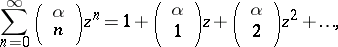whereis an integer andis an arbitrary fixed number (in general, a complex number),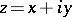is a complex variable, and the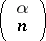are the binomial coefficients. For an integerthe binomial series reduces to a finite sum of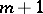terms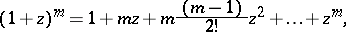which is known as the Newton binomial. For other values ofthe binomial series converges absolutely forand diverges for. At points of the unit circlethe binomial series behaves as follows: 1) if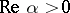, it converges absolutely at all points; 2) if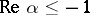, it diverges at all points; and 3) if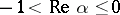, the binomial series diverges at the point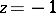and converges conditionally at all other points. At all points of convergence, the binomial series represents the principal value of the functionwhich is equal to one at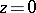. The binomial series is a special case of a hypergeometric series.

Ifandare real numbers, andis not a non-negative integer, the binomial series behaves as follows: 1) if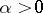, it converges absolutely on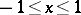; 2) if, it converges absolutely in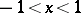and diverges at all other values of; and 3) if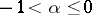, the binomial series converges absolutely in, converges conditionally at, and diverges for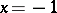; forthe binomial series always diverges.

Binomial series were probably first mentioned by I. Newton in 1664–1665. An exhaustive study of binomial series was conducted by N.H. Abel , and was the starting point of the theory of complex power series.

How to Cite This Entry:
Binomial series. Encyclopedia of Mathematics. URL: http://encyclopediaofmath.org/index.php?title=Binomial_series&oldid=17445
This article was adapted from an original article by E.D. Solomentsev (originator), which appeared in Encyclopedia of Mathematics - ISBN 1402006098. See original article